Source data

Did you write out the results of your work at the end of the last exercise? I did! We can load both source tables:

Simple hypothesis testing

At the end of the last exercise I pointed you towards two functions that perform simple hypothesis testing procedures: t.test (Student’s t-test) and aov (ANOVA). What are these procedures and how does hypothesis testing work?

Let’s start by parsing the crowd sourced answer: the first sentences on the Wikipedia pages for each.

A t-test is any statistical hypothesis test in which the test statistic follows a Student’s t-distribution if the null hypothesis is supported. It can be used to determine if two sets of data are significantly different from each other, and is most commonly applied when the test statistic would follow a normal distribution if the value of a scaling term in the test statistic were known.

R has functions that return values from all of the commonly used statistical distributions (and many uncommon ones as well!). The help page for the Student t distribution summarizes the common set of functions you’ll find for most distributions:

?TDist

The naming convention is: dxis the density function, px the probability or distribution function, qx the quantile function and rx is a random sampling function, where x is the given distribution (in this case t).

For those who have never looked at the Students t distribution of values, we can plot a sample:

plot( dt(-10:10, 1) )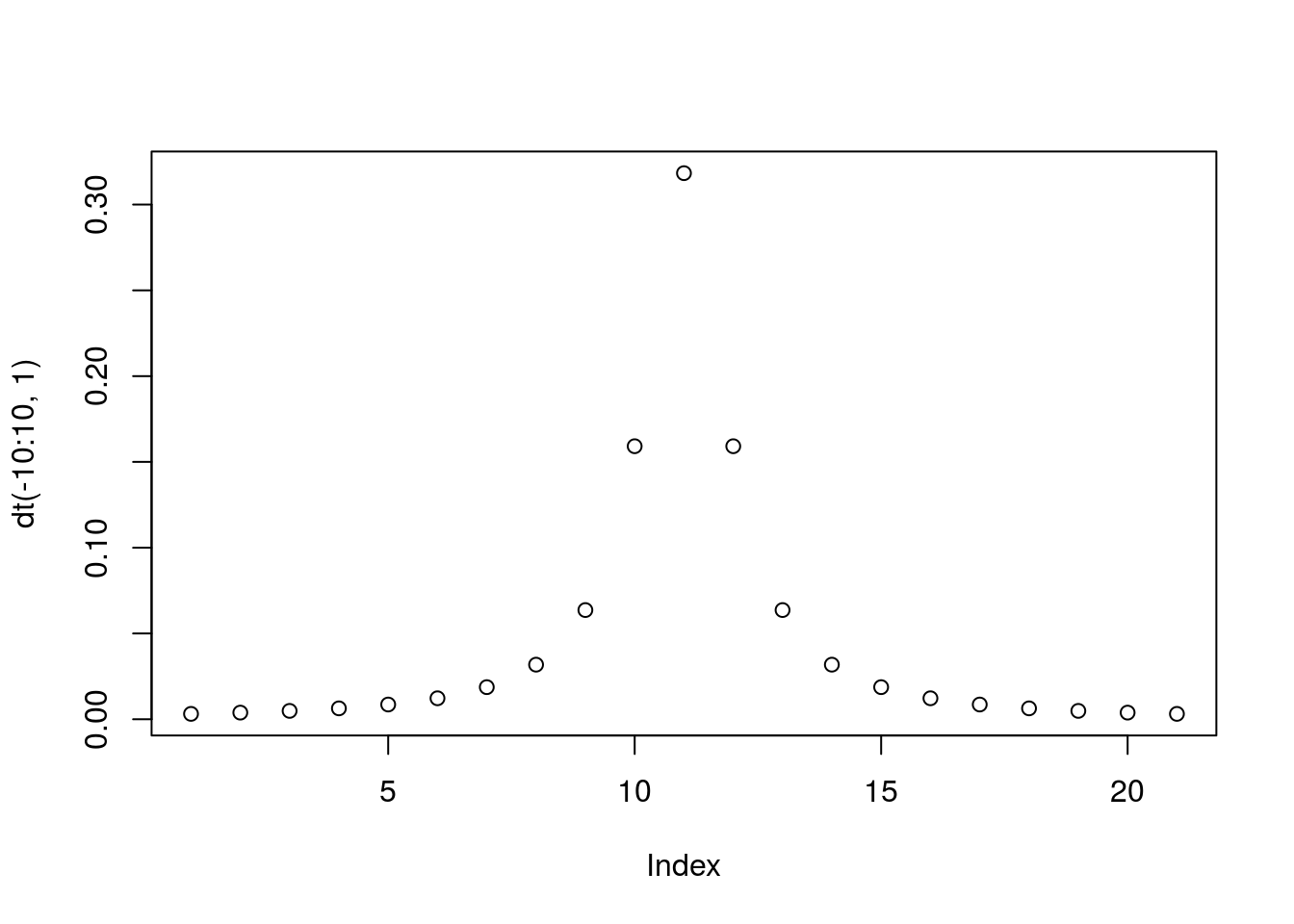Analysis of variance (ANOVA) is a collection of statistical models used to analyze the differences among group means and their associated procedures (such as “variation” among and between groups), developed by statistician and evolutionary biologist Ronald Fisher.

Fire up the ANOVA Playground app and we’ll talk about how these tests work.

Expressing hypotheses with formulas

We’ve taken a sneak peak at the formula syntax in R with the plot function, but as a reminder the basic syntax for describing a model is:

A ~ B

Where A is the dependant or response variable and B is the independant or predictor variable. More properly this form can be read as the model: A is a function of B.

Naturally, R hypothesis testing functions will take model expressions as their input (it’s why R has them). For example, if we wanted to test the following model using our tidy version of the Johnson lab data:

Average task time (ObjectAve) is a function of task complexity (Complexity)

We could use this formula syntax in a call to t.test:

t.test(ObjectAve ~ Complexity, data = jdTidy)
##
##  Welch Two Sample t-test
##
## data:  ObjectAve by Complexity
## t = -4.4255, df = 337.69, p-value = 1.3e-05
## alternative hypothesis: true difference in means is not equal to 0
## 95 percent confidence interval:
##  -1.0053871 -0.3866584
## sample estimates:
## mean in group complex  mean in group simple
##              4.397727              5.093750

Interesting! It’s always good to look at a picture to sanity check the test you just ran. Since we’re modeling a quantitative variable as a function of a categorical variable, a boxplot is a good choice:

boxplot(ObjectAve ~ Complexity, data = jdTidy)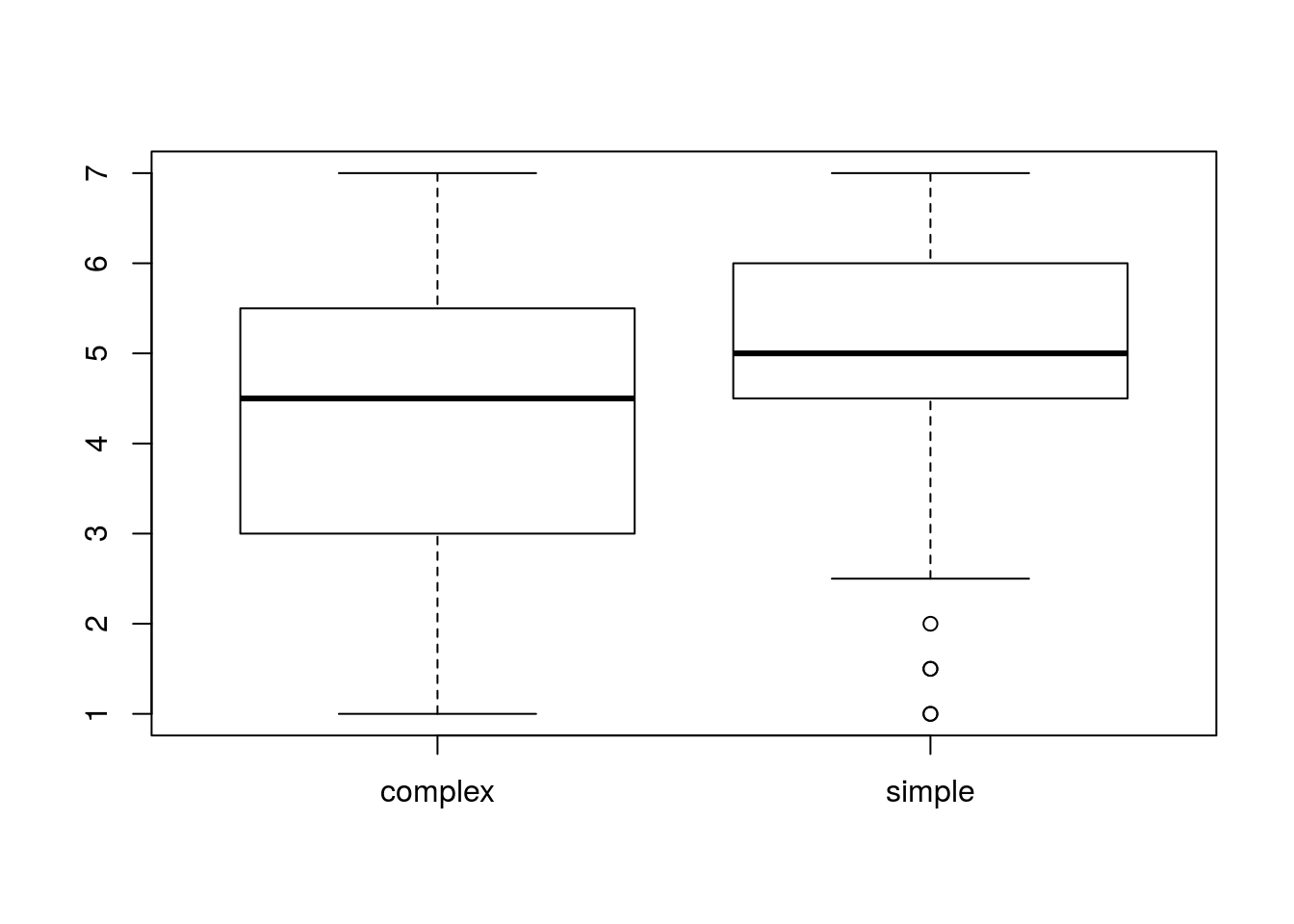We can use the aov function to test the same model using an ANOVA. The ANOVA function retuns a more complex value that t-test (which includes the regression model in addition to test results), so we’ll need to call summary to see F-statistic and related p-value:

test <- aov(ObjectAve ~ Complexity, data = jdTidy)
summary(test)
##              Df Sum Sq Mean Sq F value   Pr(>F)
## Complexity    1   42.6   42.63   19.59 1.29e-05 ***
## Residuals   350  761.9    2.18
## ---
## Signif. codes:  0 '***' 0.001 '**' 0.01 '*' 0.05 '.' 0.1 ' ' 1

In this case we would expect the ANOVA to give us a very similar prediction to the t-test. For fun, try running this:

plot(test)

The plot function is magic!

The ANOVA procedure allows you to test more complex models than a standard t-test. We can model task time as a function of a variable with multiple groups, like Education:

summary(aov(ObjectAve ~ Education, data = jdTidy))
##              Df Sum Sq Mean Sq F value Pr(>F)
## Education     6   13.6   2.264   0.994  0.429
## Residuals   343  780.7   2.276
## 2 observations deleted due to missingness
boxplot(ObjectAve ~ Education, data = jdTidy)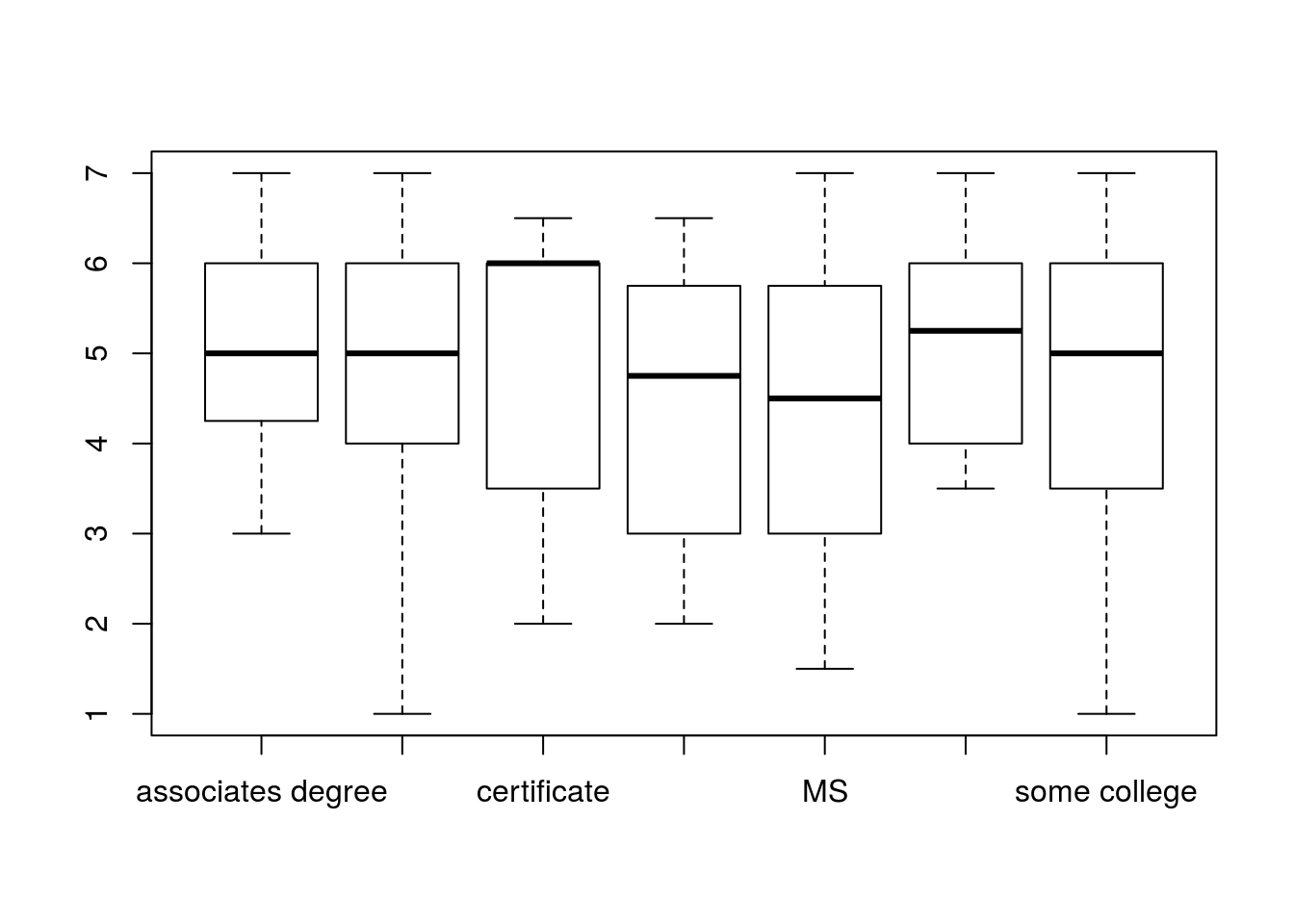You can use + to add predictors to a model. For example, if we hypothesized that both the complexity of the task and the gender of the participant contribute to the task time:

summary(aov(ObjectAve ~ Complexity + Gender, data = jdTidy))
##              Df Sum Sq Mean Sq F value   Pr(>F)
## Complexity    1   42.6   42.63  19.953 1.07e-05 ***
## Gender        1   16.2   16.20   7.582   0.0062 **
## Residuals   349  745.7    2.14
## ---
## Signif. codes:  0 '***' 0.001 '**' 0.01 '*' 0.05 '.' 0.1 ' ' 1

In some cases you might hypothesis that not only do two predictor variables influence a response variable, but that they interact (have a non-additive effect on the response). You can use : to specify an interaction. For example:

summary(aov(ObjectAve ~ Complexity:Gender, data = jdTidy))
##                    Df Sum Sq Mean Sq F value   Pr(>F)
## Complexity:Gender   3   62.7  20.903   9.807 3.17e-06 ***
## Residuals         348  741.8   2.132
## ---
## Signif. codes:  0 '***' 0.001 '**' 0.01 '*' 0.05 '.' 0.1 ' ' 1

Here’s the same along side the previous models:

summary(aov(ObjectAve ~ Complexity + Gender + Complexity:Gender, data = jdTidy))
##                    Df Sum Sq Mean Sq F value   Pr(>F)
## Complexity          1   42.6   42.63   20.00 1.05e-05 ***
## Gender              1   16.2   16.20    7.60  0.00615 **
## Complexity:Gender   1    3.9    3.88    1.82  0.17821
## Residuals         348  741.8    2.13
## ---
## Signif. codes:  0 '***' 0.001 '**' 0.01 '*' 0.05 '.' 0.1 ' ' 1

Because this is a common formulation, you can use a * to do the same:

summary(aov(ObjectAve ~ Complexity * Gender, data = jdTidy))
##                    Df Sum Sq Mean Sq F value   Pr(>F)
## Complexity          1   42.6   42.63   20.00 1.05e-05 ***
## Gender              1   16.2   16.20    7.60  0.00615 **
## Complexity:Gender   1    3.9    3.88    1.82  0.17821
## Residuals         348  741.8    2.13
## ---
## Signif. codes:  0 '***' 0.001 '**' 0.01 '*' 0.05 '.' 0.1 ' ' 1

Let’s look at a picture to see what’s going on there:

boxplot(ObjectAve ~ Complexity * Gender, data = jdTidy)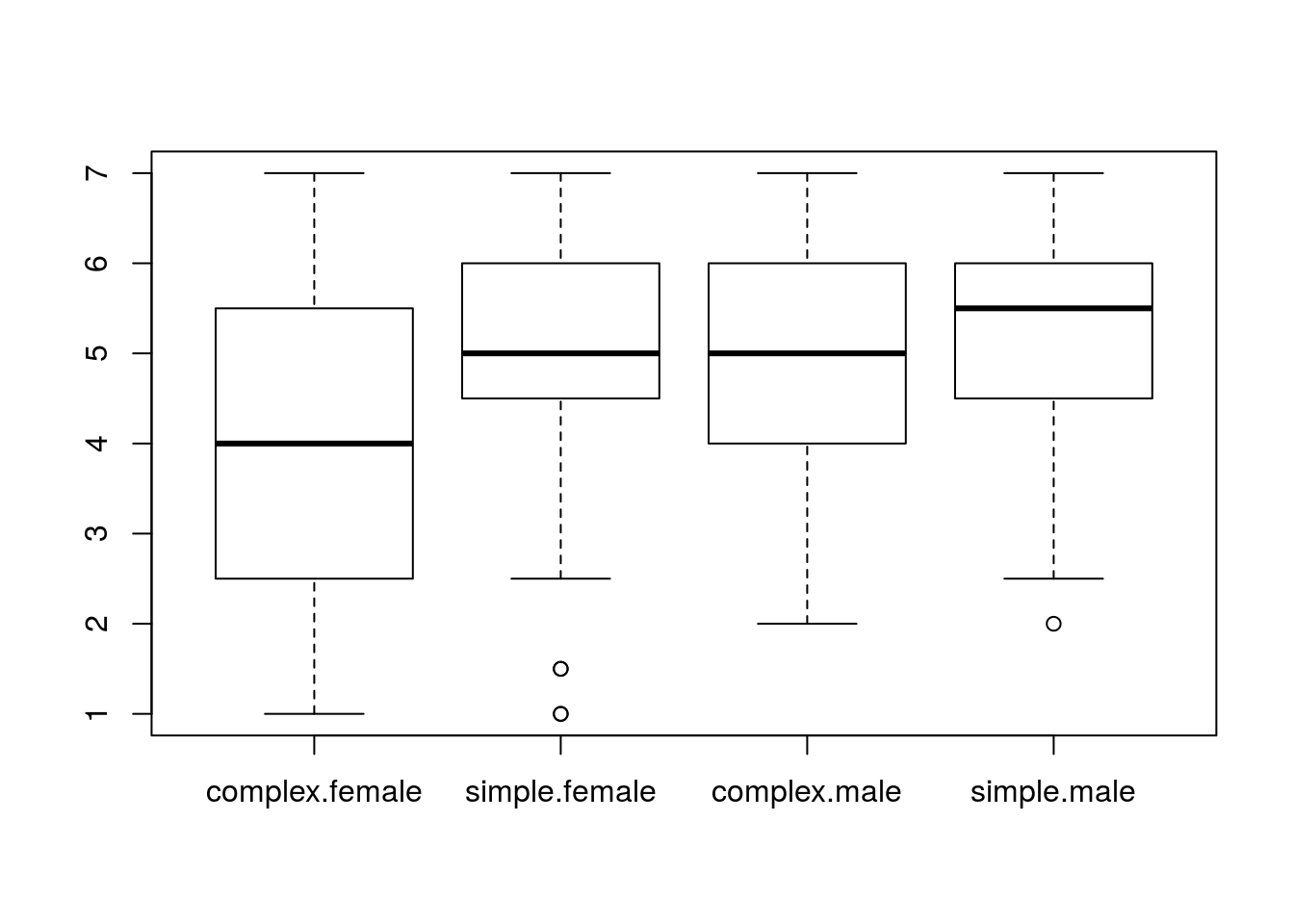See the help page for formula for additional syntax that can be used in formula expressions.

Prove to yourself that you followed along above by testing a couple more interactions in this data set:

# Enter your code here!

Regression

Although sometimes conflated, the ANOVA procedure is independant of the underlying regression model it’s being used to evaulate. If you look at the help page for the aov function, you’ll see it has been running a linear regression for us using lm. If you need to run an ANOVA with finer grain control over the regression use anova instead.

Of course you can use the lm function with a formula directly to perform the regression yourself. Here’s a plot we looked at after loading up the original data set:

plot(VelcroTime ~ VacuumTime, data = jdFact)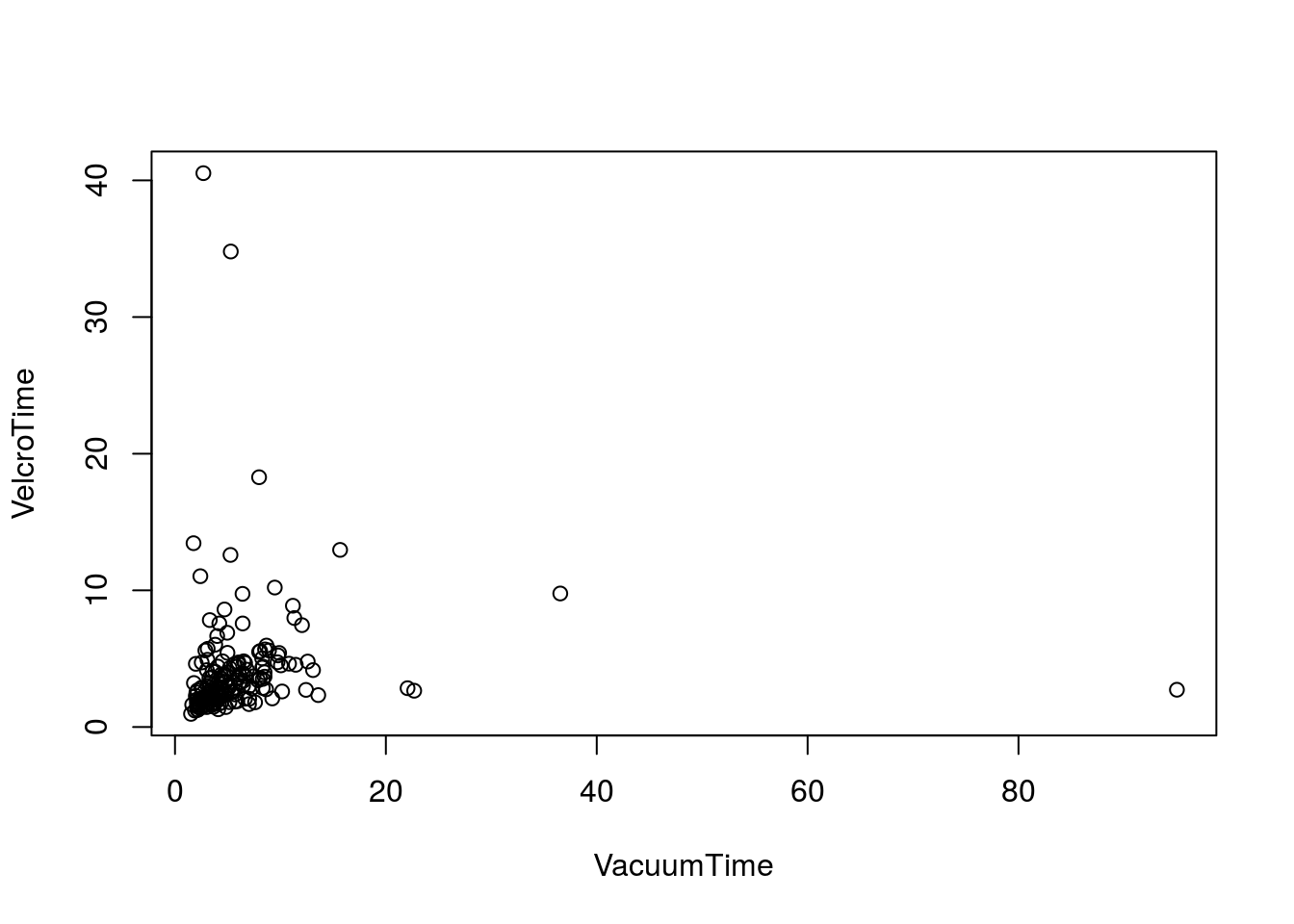The outliers in the data make it difficult to visually assess correlation between these two variables. Let’s see what we get if we fit a linear model to the data:

fit <- lm(VelcroTime ~ VacuumTime, data = jdFact)
fit
##
## Call:
## lm(formula = VelcroTime ~ VacuumTime, data = jdFact)
##
## Coefficients:
## (Intercept)   VacuumTime
##     3.88202      0.03299

For a simple linear model, the two model coefficients are probably familiar to you as the “y-intercept”" and the “slope”. You can extract the coefficients of a model into a numeric vector using coefficients:

cf <- coefficients(fit)
cf
## (Intercept)  VacuumTime
##  3.88201636  0.03298668

As was the case for the aov function, summary will provide more details about the result of fitting a linear model:

summary(fit)
##
## Call:
## lm(formula = VelcroTime ~ VacuumTime, data = jdFact)
##
## Residuals:
##    Min     1Q Median     3Q    Max
## -4.287 -1.931 -1.090  0.359 36.561
##
## Coefficients:
##             Estimate Std. Error t value Pr(>|t|)
## (Intercept)  3.88202    0.42088   9.224   <2e-16 ***
## VacuumTime   0.03299    0.04239   0.778    0.437
## ---
## Signif. codes:  0 '***' 0.001 '**' 0.01 '*' 0.05 '.' 0.1 ' ' 1
##
## Residual standard error: 4.409 on 174 degrees of freedom
## Multiple R-squared:  0.003469,   Adjusted R-squared:  -0.002258
## F-statistic: 0.6057 on 1 and 174 DF,  p-value: 0.4375

We can plot the fitted model on top of our scatter plot using abline:

plot(VelcroTime ~ VacuumTime, data = jdFact)
abline(reg = fit)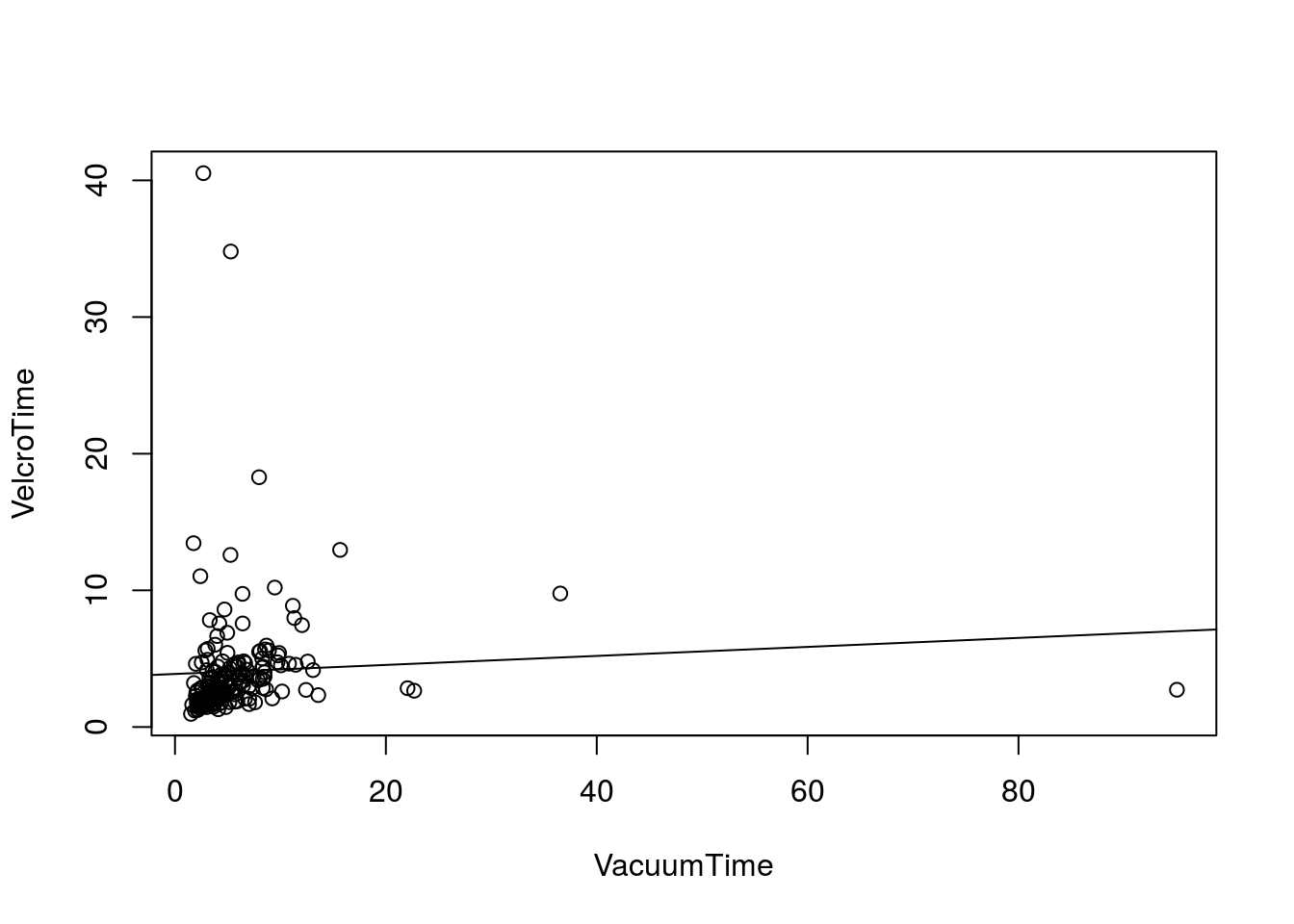The formula syntax is extremely expressive. For example, if we wanted to model logarithmic relationship using linear modeling we could use an expression like this (although this is a STUPID idea for this data):

lm(VelcroTime ~ log(VacuumTime), data = jdFact)
##
## Call:
## lm(formula = VelcroTime ~ log(VacuumTime), data = jdFact)
##
## Coefficients:
##     (Intercept)  log(VacuumTime)
##           2.513            1.002

After class

• Read Goffeau et al. 1996
• Make sure you have a working solution to the translation problem at the end of Working with Tables exercise (#2).